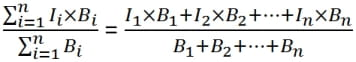School Search Form

# Calculating the Weighted Average Interest Rate

The interest rate on a federal consolidation loan is based on the weighted average of the interest rates on the federal student loans that are combined into the consolidation loan. The weighted average combines the interest rates into a single interest rate that yields a combined cost that is about the same as the cost of the original separate loans. Follow the example below to calculate the weighted average interest rate for a federal loan consolidation. This example assumes that there are three loans, each with a different interest rate.

Could student loan refinancing save you money?
loan balance and interest rate examples

Loan Balance Interest Rate
Loan #1 \$20,000 6.80%
Loan #2 \$10,000 7.90%
Loan #3 \$10,000 5.41%

## Step 1

Multiply each loan amount by its interest rate to obtain the per loan weight factor.

\$20,000 * 6.80% = \$1,360

\$10,000 * 7.90% = \$790

\$10,000 * 5.41% = \$541

## Step 2

Add the per loan weight factors together to obtain the total per loan weight factor.

\$1,360 + \$790 + \$541 = \$2,691

## Step 3

Add the loan amounts together to obtain the total loan amount.

\$20,000 + \$10,000 + \$10,000 = \$40,000

## Step 4

Divide the total per loan weight factor by the total loan amount. Multiply this by 100 to express it as a percentage. This will give you the actual interest rate.

\$2,691 / \$40,000 = 6.7275%

## Step 5

Then round the result to the next nearest 1/8%.

6.7275% rounded up to the nearest 1/8% = 6.75%

Thus the interest rate on the consolidation loan that combined these three loans would then be 6.75%.

Notice that this interest rate is above the lowest interest rate and below the highest interest rate. The weighted average interest rate is always between the highest and lowest interest rates.

## Mathematical Background

Let I1, I2, …, In be the interest rates on n loans with balances B1, B2, …, Bn. The weighted average interest rate is